Courses

# Boundary Layer Theory - 1

## 10 Questions MCQ Test Mock Test Series for Civil Engineering (CE) GATE 2020 | Boundary Layer Theory - 1

Description
This mock test of Boundary Layer Theory - 1 for Civil Engineering (CE) helps you for every Civil Engineering (CE) entrance exam. This contains 10 Multiple Choice Questions for Civil Engineering (CE) Boundary Layer Theory - 1 (mcq) to study with solutions a complete question bank. The solved questions answers in this Boundary Layer Theory - 1 quiz give you a good mix of easy questions and tough questions. Civil Engineering (CE) students definitely take this Boundary Layer Theory - 1 exercise for a better result in the exam. You can find other Boundary Layer Theory - 1 extra questions, long questions & short questions for Civil Engineering (CE) on EduRev as well by searching above.
QUESTION: 1

### A fluid with kinematic viscosity v flows in laminar stage along a flat plate with free-stream velocity V. At a distance x from the leading edge, the Reynolds number of the flow is given by R = Vx/v. The thickness of the boundary layer at x will be proportional to

Solution: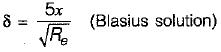QUESTION: 2

### Magnus effect may be used advantageously in games such as cricket, tennis, table tennis and golf. In order to obtain a lift, i.e. a rising curve for the trajectory of the ball, from left to right, the nature of the spin to be given is

Solution:

For rising curve, lift force in the vertical plane is needed. So for lift to right trajectory clockwise rotation should be given in horizontal plane.

QUESTION: 3

### Match List - I with List - ll and select the correct answer using the codes given below the lists: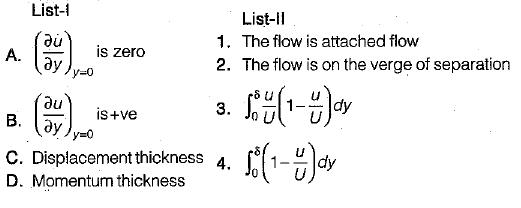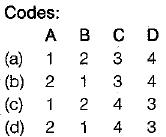Solution:

The point of separation is defined as the limit between forward arid reverse flow in the layer very close to the wall,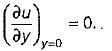The minimum pressure occur little upstream of stagnation point.

QUESTION: 4

A thin smooth plate 1 m wide and 2 m long is towed through water at a velocity of 2 m/s. Assuming that boundary remains laminar, then drag on both sides of the plate is (kinematic viscosity = 10-6 m2/s)

Solution: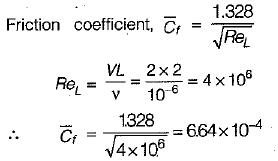The drag force on both sides of the plate,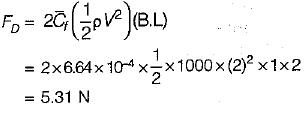QUESTION: 5

For a turbulent boundary layer (under zero pressure gradient), the velocity profile is described by the one-fifth power law. What is the ratio of displacement thickness to boundary layer thickness?

Solution: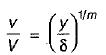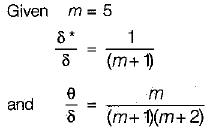δ is boundary layer thickness
δ* is displacement thickness
θ is momentum thickness Shape factor,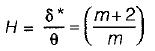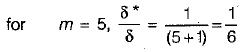QUESTION: 6

The velocity distribution for flow over a plate is given by u = 0.5y - y2 where ‘u’ is the velocity in m/s at a distance ‘y’ meter above the plate. If the dynamic viscosity of the fluid is 0.9 N-s/m2, then what is the shear stress at 0.20 m from the boundary?

Solution: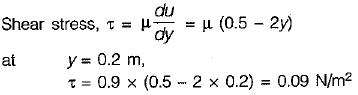QUESTION: 7

In the case of a sphere placed in a fluid stream

Solution:

For a well streamlined body the separation occurs only at the downstream end. As such the wake in this case is extremely small. Flence the pressure drag of such objects is very small fraction of that of the disc. However, the frictional drag of streamlined bodies is considerably large than that of the sphere, since there being more surface area in contact with the flow. Furthermore, for well streamlined objects friction drag is usually larger than pressure drag, but both are so small that their total drag is only about one-fortieth (1/40) of that of the disc.

QUESTION: 8

A very tiny sphere is settling down in a viscous liquid at Reynold’s number = 0.2. Its drag coefficient will be

Solution: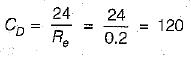QUESTION: 9

According to Prandtl-Blassius relation, the thickness of boundary layer in turbulent flow is
where x = distance between leading edge of the body and the section where thickness of boundary layer is required.
Rex = Reynold’s number at a distance x from the leading edge.

Solution:
QUESTION: 10

In which of the following the friction drag is generally larger than pressure drag?

Solution: